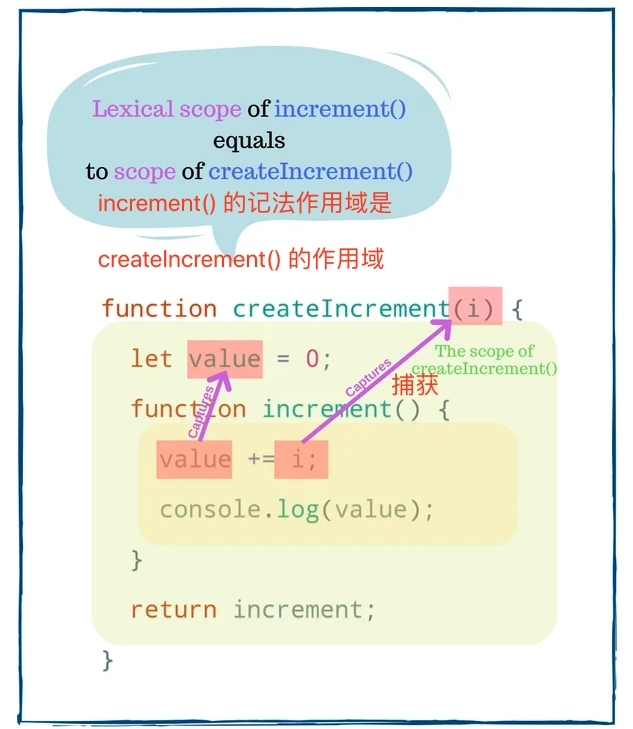# 使用js和React Hook时的过时闭包问题及解决方案

javascript

React

Hook## 1. JS 中的闭包

``````function createIncrement(i) {
let value = 0;
function increment() {
value += i;
console.log(value);
}
return increment;
}

const inc = createIncrement(1);
inc(); // 1
inc(); // 2
``````

`createIncrement(1)` 返回一个增量函数，该函数赋值给`inc`变量。当调用`inc()`时，`value` 变量加`1```````(function() {
inc(); // 3
}());

setTimeout(function() {
inc(); // 4
}, 1000);
``````

## 2. React Hooks 中的闭包

Hooks 严重依赖于 JS 闭包,但是闭包有时很棘手。

## 3. 过时的闭包

``````function createIncrement(i) {
let value = 0;
function increment() {
value += i;
console.log(value);
const message = `Current value is \${value}`;
return function logValue() {
console.log(message);
};
}

return increment;
}

const inc = createIncrement(1);
const log = inc(); // 打印 1
inc();             // 打印 2
inc();             // 打印 3
// 无法正确工作
log();             // 打印 "Current value is 1"
``````

`log()`是过时的闭包。在第一次调用 `inc()` 时，闭包 `log()` 捕获了具有 `“Current value is 1”``message` 变量。而现在，当 `value` 已经是 `3` 时，`message` 变量已经过时了。

## 4.修复过时闭包的问题

#### 使用新的闭包

``````const inc = createIncrement(1);

inc();  // 打印 1
inc();  // 打印 2
const latestLog = inc(); // 打印 3
// 正常工作
latestLog(); // 打印 "Current value is 3"
``````

`latestLog` 捕获的 `message` 变量具有最新的的值 “Current value is 3”。

Hooks 实现假设在组件重新渲染之间，作为 Hook 回调提供的最新闭包(例如 `useEffect(callback)`) 已经从组件的函数作用域捕获了最新的变量。

#### 关闭已更改的变量

``````function createIncrementFixed(i) {
let value = 0;
function increment() {
value += i;
console.log(value);
return function logValue() {
const message = `Current value is \${value}`;
console.log(message);
};
}

return increment;
}

const inc = createIncrementFixed(1);
const log = inc(); // 打印 1
inc();             // 打印 2
inc();             // 打印 3
// 正常工作
log();             // 打印 "Current value is 3"
``````

`logValue()` 关闭 `createIncrementFixed()` 作用域内的 `value` 变量。`log()` 现在打印正确的消息“`Current value is 3`”。

## 5. Hook 中过时的闭包

#### useEffect()

``````function WatchCount() {
const [count, setCount] = useState(0);

useEffect(function() {
setInterval(function log() {
console.log(`Count is: \${count}`);
}, 2000);
}, []);

return (
<div>
{count}
<button onClick={() => setCount(count + 1) }>
加1
</button>
</div>
);
}
``````

``````function WatchCount() {
const [count, setCount] = useState(0);

useEffect(function() {
const id = setInterval(function log() {
console.log(`Count is: \${count}`);
}, 2000);
return function() {
clearInterval(id);
}
}, [count]); // 看这里，这行是重点

return (
<div>
{count}
<button onClick={() => setCount(count + 1) }>
Increase
</button>
</div>
);
}
``````

#### useState()

• 点击按键 “Increase async” 在异步模式下以`1`秒的延迟递增计数器
`````` 在同步模式下，点击按键 “Increase sync” 会立即增加计数器。
function DelayedCount() {
const [count, setCount] = useState(0);
function handleClickAsync() {
setTimeout(function delay() {   setCount(count + 1); }, 1000);
}
function handleClickSync() {
setCount(count + 1);
}
return (
<div>   {count}   <button onClick={handleClickAsync}>Increase async</button>   <button onClick={handleClickSync}>Increase sync</button> </div>
);
}

``````

1. 初始渲染：`count` 值为 `0`
2. 点击 'Increase async' 按钮。`delay()` 闭包捕获 `count` 的值 `0``setTimeout()` 1 秒后调用 `delay()`
3. 点击 “Increase async” 按键。`handleClickSync()` 调用 `setCount(0 + 1)``count` 的值设置为 `1`，组件重新渲染。
4. `1` 秒之后，`setTimeout()` 执行 `delay()` 函数。但是 `delay()` 中闭包保存 `count` 的值是初始渲染的值 `0`，所以调用 `setState(0 + 1)`，结果`count`保持为 `1`

`delay()` 是一个过时的闭包，它使用在初始渲染期间捕获的过时的 `count` 变量。

``````function DelayedCount() {
const [count, setCount] = useState(0);

function handleClickAsync() {
setTimeout(function delay() {
setCount(count => count + 1); // 这行是重点
}, 1000);
}

function handleClickSync() {
setCount(count + 1);
}

return (
<div>
{count}
<button onClick={handleClickAsync}>Increase async</button>
<button onClick={handleClickSync}>Increase sync</button>
</div>
);
}
``````